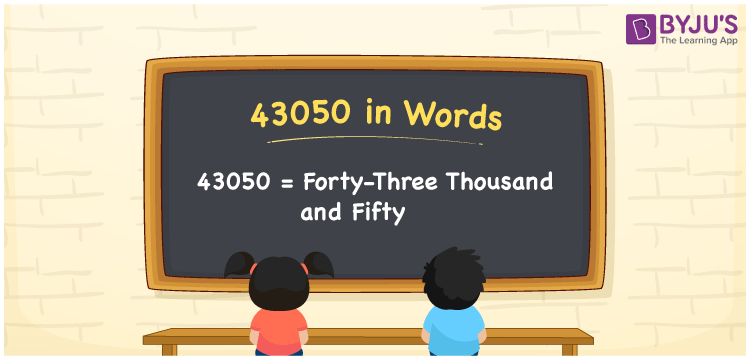# 43050 in Words

Suppose you have seen a LED TV price tag, Rs. 43050; you may read it as, “The price of a LED TV is Forty-three thousand and fifty rupees”. Thus, ee can write 43050 in words as Forty-three thousand and fifty. Let’s understand how to convert the cardinal number 43050 into words with the help of a place value chart here in this article.

 43050 in words Forty-three thousand and fifty Forty-three thousand and fifty in Numbers 43050

## 43050 in English words

Generally, we express numbers in words using the English alphabet. So, we can read 43050 in English as “Forty-three thousand and fifty”.## How to Write 43050 in Words?

As we can see, 43050 is a five-digit number, so we need to make a five-column place value chart as given below.

 Ten thousands Thousands Hundreds Tens Ones 4 3 0 5 0

Here, ones = 0, tens = 5, hundreds = 0, thousands = 3, ten thousands = 4

The above digits can be expanded as follows.

4 × Ten thousand + 3 × Thousand + 0 × Hundred + 5 × Ten + 0 × One

= 4 × 10000 + 3 × 1000 + 0 × 100 + 5 × 10 + 0 × 1

= 40000 + 3000 + 50

= Forty thousand + Three thousand + Fifty

= Forty-three thousand and fifty

Thus, 43050 in words = Forty-three thousand and fifty.

43050 is a natural number that is the successor of 43049 and the predecessor of 43051.

43050 in words – Forty-three thousand and fifty

Is 43050 an odd number? – No

Is 43050 an even number? – Yes

Is 43050 a perfect square number? – No

Is 43050 a perfect cube number? – No

Is 43050 a prime number? – No

Is 43050 a composite number? – Yes

## Frequently Asked Questions on 43050 in Words

Q1

### How do you write 43050 in words?

The number 43050 can be written in words as Forty-three thousand and fifty.
Q2

### How to write Rs. 43050 in words on a cheque?

On a cheque, we can write Rs. 43050 in words as “Forty-three thousand and fifty rupees only”.
Q3

### Write the value of 43050 + 13950 in words.

43050 + 13950 = 57000 Thus, we can write the value 43050 + 13950, i.e. 57000 in words as Fifty-seven thousand.